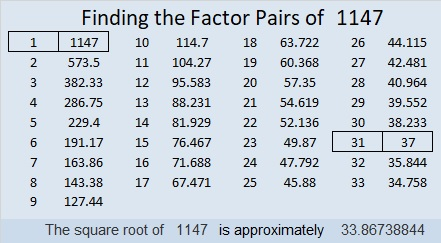# 1147 Dragonfly Challenge

This “dragonfly” challenges you to solve the puzzle. It won’t be easy, but if you stick to using logic the entire time, you will be able to do it!Print the puzzles or type the solution in this excel file: 12 factors 1134-1147

Here are a few facts about the number 1147:

• 1147 is a composite number.
• Prime factorization: 1147 = 31 × 37
• The exponents in the prime factorization are 1 and 1. Adding one to each and multiplying we get (1 + 1)(1 + 1) = 2 × 2 = 4. Therefore 1147 has exactly 4 factors.
• Factors of 1147: 1, 31, 37, 1147
• Factor pairs: 1147 = 1 × 1147 or 31 × 37
• 1147 has no square factors that allow its square root to be simplified. √1147 ≈ 33.867391147 is the hypotenuse of a Pythagorean triple:
372-1085-1147 which is 31 times (12-35-37)

34² – 3² = 1147, so we are only 9 numbers away from the next perfect square.

1147 is a leg in a few Pythagorean triples including this primitive:
204-1147-1165 calculated from 2(34)(3), 34² – 3², 34² + 3²

I like the way 1147 looks when it is written in some other bases:
It’s 5151 in BASE 6 because 5(6³) + 1(6²) + 5(6) + 1(1) = 1147,
7B7 in BASE 12 (B is 11 base 10) because 7(12²) + 11(12) + 7(1) = 1147,
and VV in BASE 36 (V is 31 base 10) because 31(36) + 31(1) = 31(37) = 1147

This site uses Akismet to reduce spam. Learn how your comment data is processed.